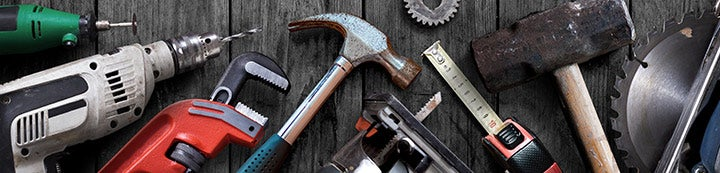1 - 3 of 3 Posts

#### veesubotee

·
##### Registered
Joined
·
299 Posts
Discussion Starter · ·
Been:

On the 'talk' forum, you replied to 'low amp draw' and asked if the WB temps were taken for supply and return to calculate actual capacity.

Could you elaborate, please, how that is done using those values?

Thanks.

V

#### beenthere

·
Joined
·
42,126 Posts
You convert wetbulb to enthapy(BTUs per pound of dry air).
Then multiply the enthapy difference by the CFM and by 4.5 to get total capacity.

EG:

Return, 76°DB 63° WB=28.477BTUs per pound dry air.
Supply, 56F DB 54°WB=22.602BTUs per pound dry air.

28.477-22.602=5.875 Enthapy Delta.
5.875Enthapy DeltaX4.5Seal Level Constant=26.437X1000CFM=26,437BTUs total capacity.

#### veesubotee

·
##### Registered
Joined
·
299 Posts
Discussion Starter · ·
You convert wetbulb to enthapy(BTUs per pound of dry air).
Then multiply the enthapy difference by the CFM and by 4.5 to get total capacity.

EG:

Return, 76°DB 63° WB=28.477BTUs per pound dry air.
Supply, 56F DB 54°WB=22.602BTUs per pound dry air.

28.477-22.602=5.875 Enthapy Delta.
5.875Enthapy DeltaX4.5Seal Level Constant=26.437X1000CFM=26,437BTUs total capacity.
Thanks, Been

1 - 3 of 3 Posts